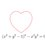# [MOOC] Introduction to Programming in HaskellI am volunteering as the teaching assistant of a MOOC in Introduction to Programming in Haskell. I'd be glad to see members from the Brilliant community join.

• Haskell is a functional language. This means, problems in Haskell are solved by describing what the problem is and how it can be solved by composing smaller logical units, called functions, together. This is as opposed to the usual sense of programming where programs are a bunch of instructions.

Consider the following implementation of Quicksort in Haskell:

 1 2 3 4 5 6 quicksort :: Ord a => [a] -> [a] quicksort [] = [] quicksort (p:xs) = (quicksort lesser) ++ [p] ++ (quicksort greater) where lesser = filter (< p) xs greater = filter (>= p) xs 

• Haskell is lazy. Haskell only evaluates an expression when needed. This means we can do cool stuff like implement infinite lists.

Consider the following implementation of an infinite list of primes Sieve of Eratosthenes:

 1 2 3 primes = filterPrime [2..] where filterPrime (p:xs) = p : filterPrime [x | x <- xs, x mod p /= 0] 

• Haskell is beautiful and concise. Plus, haskell notation is designed to be similar to usual mathematical notation.

Here is an implementation of the Bisection Algorithm in Haskell:

 1 2 3 4 5 6 7 bisection :: (Float -> Float) -> Float -> Float -> Float -> Float bisection f a b eps | (abs \$ f mid) < eps = mid | f mid > 0 = bisection f a mid eps | f mid < 0 = bisection f mid b eps where mid = (a+b)/2 

And for the technically inclined,

• Haskell is statically typed. The type of every expression is known at compile time. This makes it harder for the programmer to make errors.
• Haskell has extensive support for concurrency. This is especially simpler because of the way haskell deals with side effects.
• There are a large number of haskell libraries with support for purposes ranging from networking, graphics, parsers and more.

# Are there Computer Science problems on Brilliant which have been solved by Haskell?

Yes!

Also, this note on enumerating Integer Partitions

# Can you give me a short feel of what haskell is like?

Check out this 10 minute online tutorial.

For larger snippets of code, you could use ideone

# What are the prerequisites for the course?

None; but installing the haskell interpreter helps. Alternately, you could just always use ideone

# Where can I get more details about the course?

The NPTEL Course Page3 years, 7 months ago

This discussion board is a place to discuss our Daily Challenges and the math and science related to those challenges. Explanations are more than just a solution — they should explain the steps and thinking strategies that you used to obtain the solution. Comments should further the discussion of math and science.

When posting on Brilliant:

• Use the emojis to react to an explanation, whether you're congratulating a job well done , or just really confused .
• Ask specific questions about the challenge or the steps in somebody's explanation. Well-posed questions can add a lot to the discussion, but posting "I don't understand!" doesn't help anyone.
• Try to contribute something new to the discussion, whether it is an extension, generalization or other idea related to the challenge.

MarkdownAppears as
*italics* or _italics_ italics
**bold** or __bold__ bold
- bulleted- list
• bulleted
• list
1. numbered2. list
1. numbered
2. list
Note: you must add a full line of space before and after lists for them to show up correctly
paragraph 1paragraph 2

paragraph 1

paragraph 2

[example link](https://brilliant.org)example link
> This is a quote
This is a quote
    # I indented these lines
# 4 spaces, and now they show
# up as a code block.

print "hello world"
# I indented these lines
# 4 spaces, and now they show
# up as a code block.

print "hello world"
MathAppears as
Remember to wrap math in $$ ... $$ or $ ... $ to ensure proper formatting.
2 \times 3 $2 \times 3$
2^{34} $2^{34}$
a_{i-1} $a_{i-1}$
\frac{2}{3} $\frac{2}{3}$
\sqrt{2} $\sqrt{2}$
\sum_{i=1}^3 $\sum_{i=1}^3$
\sin \theta $\sin \theta$
\boxed{123} $\boxed{123}$

Sort by:

Interesting....

- 1 year, 10 months ago

Yes, I found it interesting too! Haskell is a lovely language

- 1 year, 10 months ago

- 1 year, 10 months ago

Sorry, I do not have the powers to add Haskell in the coding environment for Brilliant. If I could, and also had the technical expertise, I would have :)

- 1 year, 10 months ago

Can we become friends?

- 1 year, 10 months ago

Sure.

Hi friend :)

- 1 year, 10 months ago

Nice! Hello Friend $\smile$

- 1 year, 10 months ago

It does not matter. We all have restrictions

- 1 year, 10 months ago

Languages are wonders.

- 1 year, 10 months ago